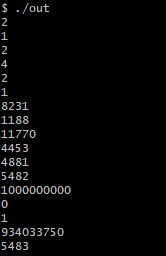# CSES Apple Division

I have coded up the following solution to CSES’ Apple Division problem. When I individually run the test cases from CSES on my local compiler, I get the correct result. But, upon submitting the problem to the online grader, all I get for each test case is a 0. Not sure why this is happening.

Here’s my code:

``````#include <bits/stdc++.h>

using namespace std;

int n, arr;
vector<long long> weightSet;
long long ans = 1e7;

void perm(int idx) {
if(idx == n) {
bool setCheck; memset(setCheck, -1, sizeof(setCheck));
long long setWeight = 0;
for(int i = 0; i < weightSet.size(); i++) {
setWeight+=arr[weightSet[i]];
setCheck[weightSet[i]] = 1;
}
long long remainingWeight = 0; for(int i = 0; i < n; i++) if(setCheck[i] != 1) remainingWeight+=arr[i];
ans = min(ans, llabs(setWeight - remainingWeight));
} else {
perm(idx+1);
weightSet.push_back(idx);
perm(idx+1);
weightSet.pop_back();
}
}

int main() {
cin >> n; for(int i = 0; i < n; i++) cin >> arr[i];

perm(0);

cout << ans << endl;
}
``````

I’ve used simple recursion to generate subsets for each group, and for each subset I am storing the minimum difference value in the `ans` variable.

Any help would be appreciated!

Have you ran the system test cases on your own machine?

Hey @SansPapyrus683,

I am not sure what you mean by system test cases, but if you are referring to the test cases that show up after submission on CSES, then yes, I have locally ran them, and they are returning the correct output. However, I cannot say the same for CSES’ OJ, and I can’t seem to figure out why this is happening.

Running it on https://ide.usaco.guide/ prints 0.

`bool`s can only be 0 or 1…

`memset`ing it -1 is undefined behavior.

@Benq, yes apparently it’s happening in https://ide.usaco.guide/ too. I have downloaded all of CSES’ test cases and ran them on my system and this is the output I am getting on my console:Each line is a the output for it’s respective test case on CSES. Could it be possible that the problem is caused by too much memory, since in each recursive step I am creating a new array?

The above output was generated using the following code:

``````#include <bits/stdc++.h>

using namespace std;

int n, arr;
vector<long long> weightSet;
long long ans = 1e10;

void perm(int idx) {
if(idx == n) {
bool weightSetCheck; memset(weightSetCheck, 0, sizeof(weightSetCheck));
long long weightSetWeight = 0;
for(int i = 0; i < weightSet.size(); i++) {
weightSetWeight+=arr[weightSet[i]];
weightSetCheck[weightSet[i]] = 1;
}
long long remainingWeight = 0; for(int i = 0; i < n; i++) if(weightSetCheck[i] != 1) remainingWeight+=arr[i];
ans = min(ans, llabs(weightSetWeight - remainingWeight));
} else {
perm(idx+1);
weightSet.push_back(idx);
perm(idx+1);
weightSet.pop_back();
}
}

void solve() {
cin >> n; for(int i = 0; i < n; i++) cin >> arr[i];

perm(0);
weightSet.clear();

cout << ans << endl;

ans = 1e10;
}

int main() {
for(int i = 1; i<=17; i++) {
freopen((string("test_input ")+"("+to_string(i)+").txt").c_str(), "r", stdin);
solve();
}
}
``````

@dongliu0426 thank you! I had totally missed that out of habit. This fixed the issue. The code passes all test cases now.